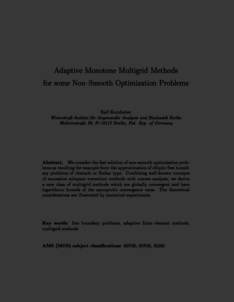Repository: Freie Universität Berlin, Math Department

Adaptive monotone multigrid methods for some non-smooth optimization problems

Kornhuber, R. (1997) Adaptive monotone multigrid methods for some non-smooth optimization problems. In: Domain decomposition methods in science and engineering: 8th international conference, Bejing, P.R. China. John Wiley & Sons, pp. 177-191. ISBN 047196560XPreview

501kB

Official URL: http://www.ddm.org/DD08/

Abstract

We consider the fast solution of non-smooth optimization problems as resulting for example from the approximation of elliptic free boundary problems of obstacle or Stefan type. Combining well-known concepts of successive subspace correction methods with convex analysis, we derive a new class of multigrid methods which are globally convergent and have logarithmic bounds of the asymptotic convergence rates. The theoretical considerations are illustrated by numerical experiments.

Item Type: Book Section Mathematical and Computer Sciences > Mathematics > Numerical Analysis Department of Mathematics and Computer Science > Institute of Mathematics 1938 Ekaterina Engel 30 Aug 2016 19:58 03 Mar 2017 14:42

Repository Staff Only: item control page